Courses

# Screw Gauge Class 11 Notes | EduRev

## Class 11 : Screw Gauge Class 11 Notes | EduRev

The document Screw Gauge Class 11 Notes | EduRev is a part of the Class 11 Course Physics Class 11.
All you need of Class 11 at this link: Class 11

SCREW GAUGE
Purpose of Screw Gauge

• To measure the diameter of the given lead shot.
• To measure the diameter of a given wire and find its volume.
• To measure the thickness of a given glass plate and find its volume.
• To measure the volume of an irregular lamina.

The Theory
The screw gauge is an instrument used for measuring accurately the diameter of a thin wire or the thickness of a sheet of metal.  It consists of a U-shaped frame fitted with a screwed spindle which is attached to a thimble.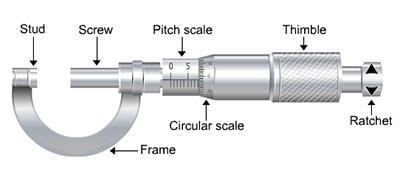Fig. Screw Gauge

Parallel to the axis of the thimble, a scale graduated in mm is engraved. This is called pitch scale. A sleeve is attached to the head of the screw.
The head of the screw has a ratchet which avoids undue tightening of the screw. On the thimble there is a circular scale known as head scale which is divided into 50 or 100 equal parts. When the screw is worked, the sleeve moves over the pitch scale.
A stud with a plane end surface called the anvil is fixed on the ‘U’ frame exactly opposite to the tip of the screw. When the tip of the screw is in contact with the anvil, usually, the zero of the head scale coincides with the zero of the pitch scale.

Pitch of the Screw Gauge
The pitch of the screw is the distance moved by the spindle per revolution. To find this, the distance advanced by the head scale over the pitch scale for a definite number of complete rotation of the screw is determined.

The pitch can be represented as;
Pitch of the screw = Distance moved by screw/No. of full rotations given  .......(1)

Least Count of the Screw Gauge
The Least count (LC) is the distance moved by the tip of the screw, when the screw is turned through 1 division of the head scale.
The least count can be calculated using the formula;
Least count = (Pitch/Total number of divisions on the circular scale) ....(2)

Zero Error and Zero Correction
To get the correct measurement, the zero error must be taken into account. For this purpose, the screw is rotated forward till the screw just touches the anvil and the edge of cap is on the zero mark of the pitch scale. The Screw gauge is held keeping the pitch scale vertical with its zero down wards.

When this is done, anyone of the following three situations can arise:
(a) The zero mark of the circular scale comes on the reference line. In this case, the zero error and the zero correction, both are nil.

(b) The zero mark of the circular scale remains above the reference line and does not cross it.  In this case, the zero error is positive and the zero correction is negative depending on how many divisions it is above the reference line.

(c) The zero mark of the head scale is below the reference line.  In this case, the zero error is negative and the zero correction is positive depending on how many divisions it is below the reference line.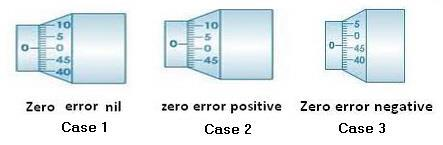To find the diameter of the lead shot with the lead shot between between the screw and anvil, if the edge of the cap lies ahead of the Nth division of the linear scale.
Then, linear scale reading (P.S.R.) = N.
If nth division of circular scale lies over reference line.
Then, circular scale reading (H.S.R.) = n × (L.C.) (L.C. is least count of screw gauge)
Total reading (T.R.) = P.S.R. + corrected H.S.R. = N + (n x L.C.).
If D be the mean diameter of lead shot,
Then, volume of the lead shot,
V = (4/3)π (D/2)3

To find the diameter and hence to calculate the volume of the wire
Place the wire between the anvil and the screw and note down the PSR and HSR as before.
The diameter of the wire is given by;
T.R = PSR + (corrected HSR × L.C) = N + (n × L.C) ....(3)

If r is radius of the wire, and l be the mean length of the wire.
Then, volume of the wire,
V = π r2 I ....(4)

To find the thickness of the glass plate
The glass plate is gripped between the tip of the screw and the anvil. The PSR and HSR are noted as before.
The thickness of the glass plate is;
t = PSR + corrected HSR = N + (n × L.C) ....(5)

To find the Volume of glass plate (irregular lamina)
Find the thickness, t of irregular lamina as before. Then place the lamina over a graph paper and trace its outline on the graph paper. The area A of the lamina is taken from the graph paper.
The volume of the glass plate is calculated from the equation;
V = A ∗ t ........ (6)

Learning Outcomes
The students learns;
(1) Different parts of the screw gauge.
(2) How to use a screw gauge.
(3) How to calculate the least count of screw gauge.
(4) How to calculate the zero error and zero correction of a screw gauge.
(5) How to calculate the volume of a lead shot by measuring its diameter.
(6) How to calculate the volume of a glass plate by measuring its thickness.
(7) How to calculate the volume of a wire by measuring its diameter.

Materials Required

• Screw gauge
• Wire
• A sheet of paper
• An irregular lamina
• A centimetre graph paper
• A pointed pencil

Real lab Procedure
1. Determine the pitch and least count of the screw gauge using the equations (1) and (2) respectively.
2. Bring the anvil and screw in contact with each other and find the zero error. Do it three times and record them. If there is no zero error, then record ‘zero error nil’.
3. Move the screw away from the anvil and place the lead shot and move the screw towards the anvil using the ratchet head. Stop when the ratchet slips without moving the screw.
4. Note the number of divisions on the pitch scale that is visible and uncovered by the edge of the cap. The reading N is called the pitch scale reading (PSR)
5. Note the number (n) of the division of the circular scale lying over the reference line.
6. Repeat steps 4 and 5 after rotating the lead shot by 900 for measuring the diameter in a perpendicular direction. Record the observations in the tabular column.
7. Find total reading using the equation 3 and apply zero correction in each case.
8. Take the mean of different values.

Note: Place the other objects like, wire, glass plate etc between the screw and the anvil and follow the above procedure to find the measurement.

Observations
1. Determination of Least Count of the Screw Gauge
1 Linear Scale Division, LSD = 1 mm
Number of full rotations given to screw = 4
Distance moved by the screw = 4 mm
Hence , pitch p = 4 mm/4 = 1 mm
Number of divisions on circular scale = 100
Hence, least count, L.C = 1mm/100 = 0.01 mm = 0.001 cm

2. Zero Error
(a) zero error = --------------mm
(b)  zero error = ---------------mm
(c) zero error = ----------------mm
Mean zero error, e = ------------mm
Mean zero correction , c = -e = -------mm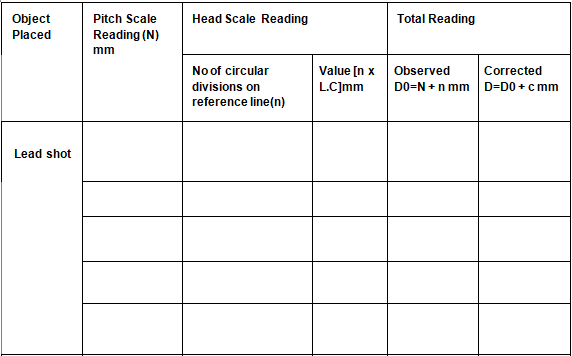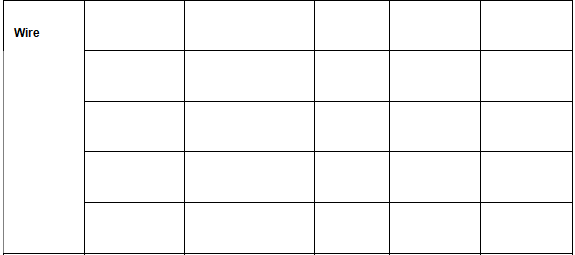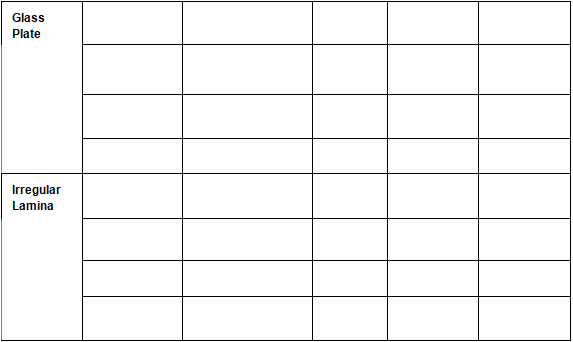Calculations
Mean Diameter of the lead shot = ---------cm
Mean Diameter of the wire = --------cm
Mean length of the wire = ---------cm
Volume of the wire, V = π(D/2)I = ---------cm3
Thickness of the glass plate = -------cm
Thickness of irregular lamina = -------cm
Area, A = -----------------cm2
Volume of irregular lamina,
V= A × t = ---------- cm
Result
Diameter of the lead shot = ----------cm
The volume of the given wire is ------ cm3
The thickness of given sheet is ----------cm
The volume of given lamina is = ------- cm3

SOLVED EXAMPLES
Example 1. What are the readings of the reading of the micrometer meter screw gauges as shown in the figures below?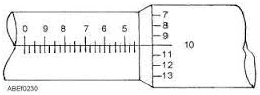SolutionReading of main scale = 4.50 mm
Reading of vernier scale = 10
Reading of Vernier scale x accuracy of Vernier caliper = 10 × 0.01 = 0.10 mm
Add the result of step III and step I to get the reading of the micrometer screw gauge.
= 4.50 + 0.10 = 4.60 mm
Reading of Vernier caliper = 4.60 mm

Example 2. What are the readings of the reading of the micrometer meter screw gauges as shown in the figures below?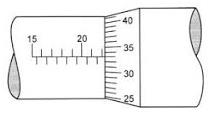Solution. Reading of main scale = 22.0 mm
Reading of Vernier scale = 33
Reading of Vernier scale x accuracy of
Vernier caliper = 33 × 0.01 = 0.33 mm
Add the result of step III and step I to get the reading of the micrometer screw gauge.
= 22.0 + 0.33 = 22.33 mm
Reading of Vernier caliper = 22.33 mm

Offer running on EduRev: Apply code STAYHOME200 to get INR 200 off on our premium plan EduRev Infinity!

,

,

,

,

,

,

,

,

,

,

,

,

,

,

,

,

,

,

,

,

,

;